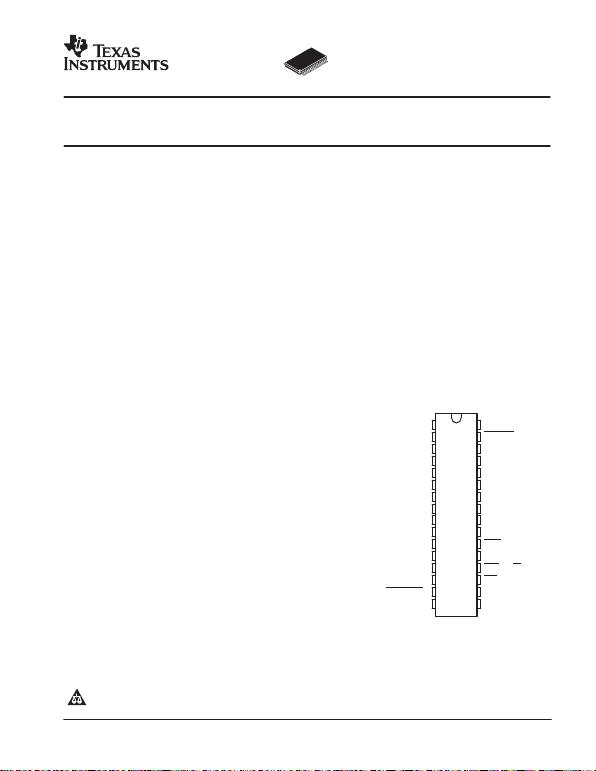
SLAS254B − MAY 2002 − REVISED NOVEMBER 2002
      
 
FEATURES
DSimultaneous Sampling of Two Single-Ended
Signals or One Differential Signal
DIntegrated 16-Word FIFO
DSignal-to-Noise and Distortion Ratio: 59 dB at
fI = 2 MHz
DDifferential Nonlinearity Error: ±1 LSB
DIntegral Nonlinearity Error: ±1 LSB
DAuto-Scan Mode for Two Inputs
D3-V or 5-V Digital Interface Compatible
DLow Power: 216 mW Max
D5-V Analog Single Supply Operation
DInternal Voltage References ...50 PPM/°C
and ±5% Accuracy
DParallel µC/DSP Interface
APPLICATIONS
DCommunications
DControl Applications
DHigh-Speed DSP Front-End
DAutomotive Applications
DESCRIPTION
The THS10082 is a CMOS, low-power, 10-bit, 8 MSPS
analog-to-digital converter (ADC). The speed, resolution,
bandwidth, and single-supply operation are suited for
applications in radar, imaging, high-speed acquisition, and
communications. A multistage pipelined architecture with
output error correction logic provides for no missing codes
over the full operating temperature range. Internal control
registers allow for programming the ADC into the desired
which are sampled simultaneously. These inputs can be
selected individually and configured to single-ended or
differential inputs. An integrated 16 word deep FIFO
allows the storage of data in order to take the load off
of the processor connected to the ADC. Internal
reference voltages for the ADC (1.5 V and 3.5 V) are
provided.
An external reference can also be chosen to suit the dc
accuracy and temperature drift requirements of the
application. Two different conversion modes can be
selected. In the single conversion mode, a single and
simultaneous conversion can be initiated by using the
single conversion start signal (CONVST). The conversion
clock in the single conversion mode is generated internally
using a clock oscillator circuit. In the continuous
conversion mode, an external clock signal is applied to the
CONV_CLK input of the THS10082. The internal clock
oscillator is switched off in the continuous conversion
mode.
The THS10082C is characterized for operation from 0°C
to 70°C, and the THS10082I is characterized for operation
from −40°C to 85°C.
1
2
3
4
5
6
7
8
9
10
11
12
13
14
15
16
32
31
30
29
28
27
26
25
24
23
22
21
20
19
18
17
D0
D1
D2
D3
D4
D5
BVDD
BGND
D6
D7
D8
D9
RA0
RA1
CONV_CLK (CONVST)
DATA_AV
OV_FL
RESET
AINP
AINM
REFIN
REFOUT
REFP
REFM
AGND
AVDD
CS0
CS1
WR (R/W
)
RD
DVDD
DGND
DA PACKAGE
(TOP VIEW)
  !" # \$%&" !#  '%()\$!" *!"&+ *%\$"#
\$ " #'&\$\$!"# '& ",& "&#  &-!# #"%&"# #"!*!* .!!"/+
*%\$" '\$&##0 *&# " &\$&##!)/ \$)%*& "&#"0  !)) '!!&"&#+
Please be aware that an important notice concerning availability, standard warranty, and use in critical applications of Texas Instruments
semiconductor products and disclaimers thereto appears at the end of this data sheet.
www.ti.com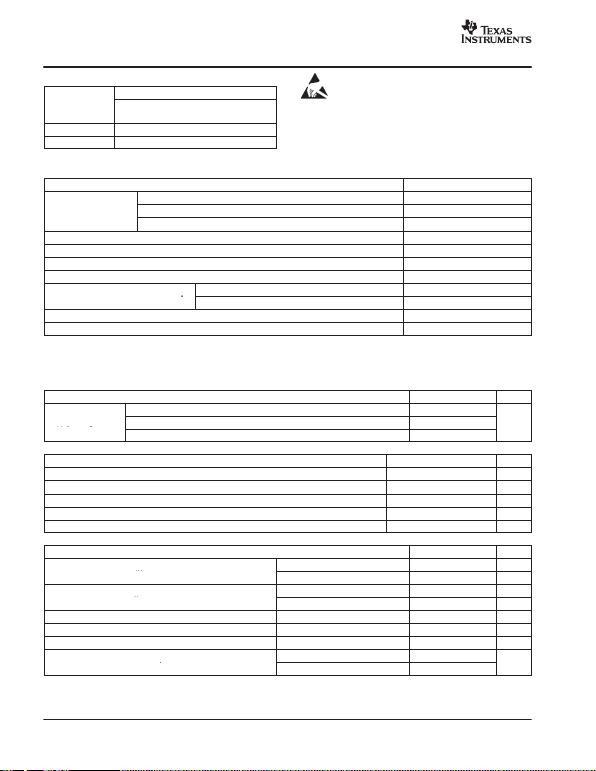
SLAS254B − MAY 2002 − REVISED NOVEMBER 2002
www.ti.com
2
ORDERING INFORMATION
PACKAGED DEVICE
TATSSOP
(DA)
0°C to 70°C THS10082CDA
−40°C to 85°C THS10082IDA
These devices have limited built-in ESD protection. The
leads should be shorted together or the device placed in
conductive foam during storage or handling to prevent
electrostatic damage to the MOS gates.
ABSOLUTE MAXIMUM RATINGS
over o p e r a t i n g f ree-air temperature range unless otherwise noted(1)
THS10082
DGND to DVDD −0.3 V to 6.5 V
Supply voltage range BGND to BVDD −0.3 V to 6.5 V
Supply voltage range
AGND to AVDD −0.3 V to 6.5 V
Analog input voltage range AGND −0.3 V to AVDD + 1.5 V
Reference input voltage −0.3 V + AGND to AVDD + 0.3 V
Digital input voltage range −0.3 V to BVDD/DVDD + 0.3 V
Operating virtual junction temperature range, TJ−40°C to 150°C
Operating free-air temperature range, TA
THS10082C 0°C to 70°C
Operating free-air temperature range, T
ATHS10082I −40°C to 85°C
Storage temperature range, Tstg −65°C to 150°C
Lead temperature 1,6 mm (1/16 inch) from case for 10 seconds 260°C
(1) Stresses beyond those listed under “absolute maximum ratings” may cause permanent damage to the device. These are stress ratings only , a nd
functional operation of the device at these or any other conditions beyond those indicated under “recommended operating conditions” is not
implied. Exposure to absolute-maximum-rated conditions for extended periods may affect device reliability.
RECOMMENDED OPERATING CONDITIONS
POWER SUPPLY MIN NOM MAX UNIT
AVDD 4.75 5 5.25
Supply voltage DVDD 3 3.3 5.25 V
Supply voltage
BVDD 3 3.3 5.25
V
ANALOG AND REFERENCE INPUTS MIN NOM MAX UNIT
Common-mode input voltage VCM in differential configuration 1 2.5 4 V
External reference voltage,VREFP (optional) 3.5 AVDD−1.2 V
External reference voltage, VREFM (optional) 1.4 1.5 V
Input voltage difference, REFP − REFM 2 V
DIGITAL INPUTS MIN NOM MAX UNIT
High-level input voltage, VIH
BVDD = 3 V 2 V
High-level input voltage, V
IH BVDD = 5.25 V 2.6 V
Low-level input voltage, VIL
BVDD = 3 V 0.6 V
Low-level input voltage, V
IL BVDD = 5.25 V 0.6 V
Input CONV_CLK frequency DVDD = 3 V to 5.25 V 0.1 8 MHz
CONV_CLK pulse duration, clock high, tw(CONV_CLKH) DVDD = 3 V to 5.25 V 62 83 5000 ns
CONV_CLK pulse duration, clock low, tw(CONV_CLKL) DVDD = 3 V to 5.25 V 62 83 5000 ns
Operating free-air temperature, TA
THS10082CDA 0 70
°C
Operating free-air temperature, T
ATHS10082IDA −40 85 °
C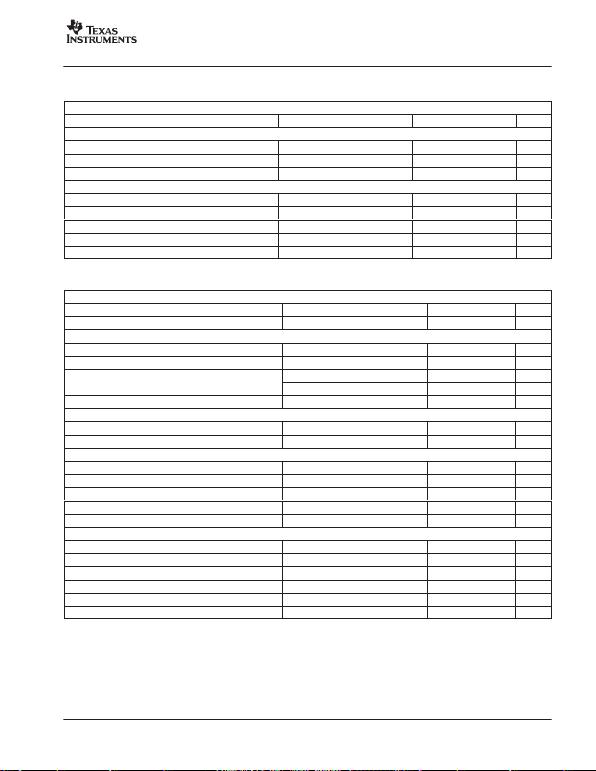
SLAS254B − M AY 2002 − REVISED NOVEMBER 2002
www.ti.com
3
ELECTRICAL CHARACTERISTICS
over recommended operating conditions, DVDD = 3.3 V, AVDD = 5 V, VREF = internal (unless otherwise noted)
DIGITAL SPECIFICATIONS
PARAMETER TEST CONDITIONS MIN TYP MAX UNIT
Digital inputs
IIH High-level input current DVDD = digital inputs −50 50 µA
IIL Low-level input current Digital input = 0 V −50 50 µA
CiInput capacitance 5 pF
Digital outputs
VOH High-level output voltage IOH = −50 µA, BVDD = 3.3 V, 5 V BVDD−0.5 V
VOL Low-level output voltage IOL = −50 µA, BVDD = 3.3 V, 5 V 0.4 V
IOZ High-impedance-state output current CS1 = DGND, CS0 = DVDD −10 10 µA
COOutput capacitance 5 pF
CLLoad capacitance at databus D0 −D9 30 pF
ELECTRICAL CHARACTERISTICS
over recommended operating conditions, A VDD = 5 V, DVDD = BVDD = 3.3 V, fs = 8 MSPS, VREF = internal (unless otherwise noted)
DC SPECIFICATIONS
PARAMETER TEST CONDITIONS MIN TYP MAX UNIT
Resolution 10 Bits
Accuracy
Integral nonlinearity, INL ±1 LSB
Differential nonlinearity, DNL ±1 LSB
Offset error
After calibration in single-ended mode ±5 LSB
Offset error
After calibration in differential mode −10 10 LSB
Gain error −10 10 LSB
Input capacitance 15 pF
Input leakage current VAIN = VREFM to VREFP ±10 µA
Internal voltage reference
VREFP Accuracy 3.3 3.5 3.7 V
VREFM Accuracy 1.4 1.5 1.6 V
Temperature coefficient 50 PPM/°C
Reference noise 100 µV
Accuracy, REFOUT 2.475 2.5 2.525 V
Power supply
IDDA Analog supply current AVDD = 5 V, BVDD = DVDD = 3.3 V 36 40 mA
IDDD Digital supply current AVDD = 5 V, BVDD = DVDD = 3.3 V 0.5 1 mA
IDDB Buffer supply current AVDD = 5 V, BVDD = DVDD = 3.3 V 1.5 4 mA
IDD_AP Analog supply current in power-down mode AVDD = 5 V, BVDD = DVDD = 3.3 V 8 mA
PDPower dissipation AVDD = 5 V, DVDD = BVDD = 3.3 V 186 216 mW
Power dissipation in powerdown AVDD = 5 V, DVDD = BVDD = 3.3 V 30 mW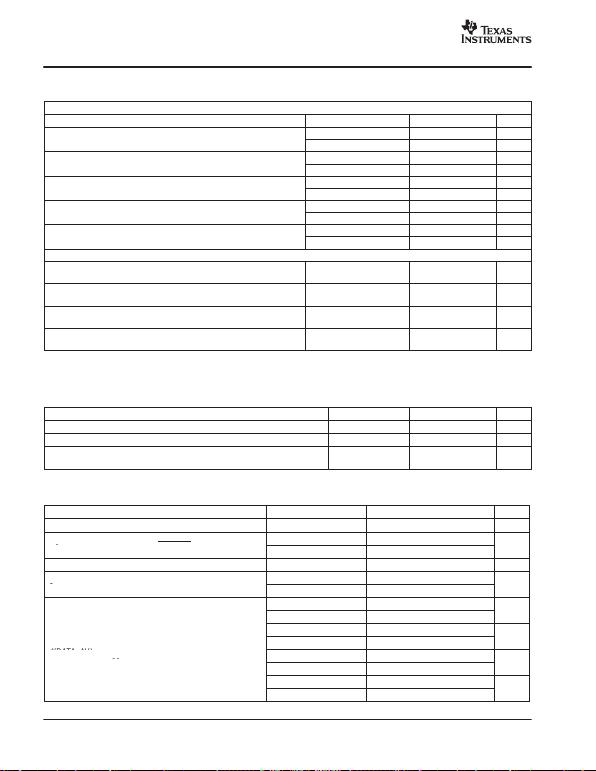
SLAS254B − M AY 2002 − REVISED NOVEMBER 2002
www.ti.com
4
ELECTRICAL CHARACTERISTICS
over recommended operating conditions, VREF = internal, fs = 8 MHz, fI = 2 MHz at −1 dB (unless otherwise noted)
AC SPECIFICATIONS, AVDD = 5 V, BVDD = DVDD = 3.3 V, CL < 30 pF
PARAMETER TEST CONDITIONS MIN TYP MAX UNIT
Differential mode 56 59 dB
Single-ended mode(1) 55 58 dB
SNR
Differential mode 59 61 dB
SNR
Single-ended mode(1) 60 dB
THD
Differential mode −67 −61 dB
THD
Single-ended mode −63 dB
ENOB
Differential mode 9 9.5 Bits
ENOB
Single-ended mode(1) 9.35 Bits
SFDR
Differential mode 61 65 dB
SFDR
Single-ended mode 64 dB
Full-power bandwidth with a source impedance of 150 in
dif ferential configuration. Full-scale sinewave, −3 dB 96 MHz
Full-power bandwidth with a source impedance of 150 in
single-ended configuration. Full-scale sinewave, −3 dB 54 MHz
Small-signal bandwidth with a source impedance of 150 in
dif ferential configuration. 100-mVpp sinewave, −3 dB 96 MHz
Small-signal bandwidth with a source impedance of 150 in
single-ended configuration. 100-mVpp sinewave, −3 dB 54 MHz
(1) The SNR (ENOB) and SINAD is degraded typically by 2 dB in single-ended mode when the reading of data is asynchronous to the sampling
clock.
TIMING REQUIREMENTS(1)
AVDD = DVDD = 5 V, BVDD = 3.3 V, VREF = internal, CL < 30 pF
PARAMETER TEST CONDITIONS MIN TYP MAX UNIT
td(DATA_AV) Delay time 5 ns
td(o) Delay time 5 ns
tpipe Latency 5 CONV
CLK
(1) See Figure 27.
TIMING SPECIFICATION OF THE SINGLE CONVERSION MODE(1)
PARAMETER TEST CONDITIONS MIN TYP MAX UNIT
tcClock cycle of the internal clock oscillator 117 125 133 ns
tw1
Pulse duration, CONVST
ns
t
w1
Pulse duration, CONVST
ns
td(A) Aperture time 1 ns
t2
Delay time between consecutive start of
ns
t
2
Delay time between consecutive start of
ns
ns
ns
ns
td(DATA_AV)
Delay time, DATA_AV becomes active for the
ns
t
d(DATA_AV)
Delay time, DATA_AV becomes active for the
trigger level condition: TRIG0 = 1, TRIG1 = 1 One analog input, TL = 8 7×t2 +6.5×tc+15
ns
trigger level condition: TRIG0 = 1, TRIG1 = 1
ns
ns
ns
(1) See Figure 26.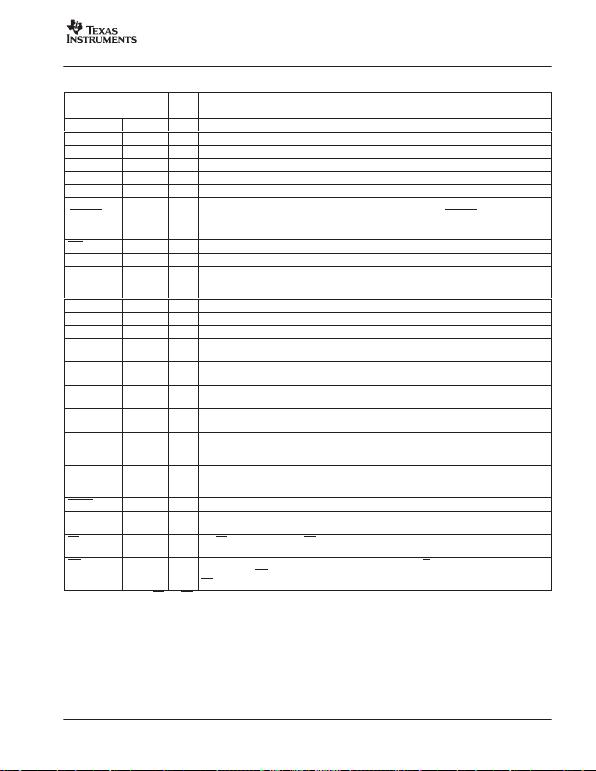
SLAS254B − M AY 2002 − REVISED NOVEMBER 2002
www.ti.com
5
Terminal Functions
TERMINAL
I/O
DESCRIPTION
NAME NO.
I/O
DESCRIPTION
AINP 30 I Analog input, single-ended or positive input of differential channel A
AINM 29 I Analog input, single-ended or negative input of differential channel A
AVDD 23 I Analog supply voltage
AGND 24 I Analog ground
BVDD 7 I Digital supply voltage for buffer
BGND 8 I Digital ground for buffer
CONV_CLK
(CONVST) 15 I Digital input. This input is used to apply an external conversion clock in the continuous conversion mode. In
the single conversion mode, this input functions as the conversion start (CONVST) input. A high-to-low
transition on this input holds simultaneously the selected analog input channels and initiates a single
CS0 22 I Chip select input (active low)
CS1 21 I Chip select input (active high)
DATA_AV 16 O Data available signal, which can be used to generate an interrupt for processors and as a level information
of the internal FIFO. This signal can be configured to be active low or high and can be configured as a static
level or pulse output. See Table 14.
DGND 17 I Digital ground. Ground reference for digital circuitry.
DVDD 18 I Digital supply voltage
D0–D9 1–6, 9–12 I/O/Z Digital input, output; D0 = LSB
RA0 13 I Digital input. RA0 is used as an address line (RA0) for the control register. This is required for writing to
control register 0 and control register 1. See Table 8.
RA1 14 I Digital input. RA1 is used as an address line (RA1) for the control register. This is required for writing to
control register 0 and control register 1. See Table 8.
OV_FL 32 O Overflow o u tput. Indicates whether an overflow in the FIFO occurred. OV_FL is set to active high level if an
overflow occurs. It is set back to low level with a reset of the THS10082 or a reset of the FIFO.
REFIN 28 I Common-mode reference input for the analog input channels. It is recommended that this pin be
connected to the reference output REFOUT.
REFP 26 I Reference input, requires a bypass capacitor of 10 µF to AGND in order to bypass the internal reference
voltage. A n e x t ernal reference voltage at this input can be applied. This option can be programmed through
control register 0. See Table 9.
REFM 25 I Reference input, requires a bypass capacitor of 10 µF to AGND in order to bypass the internal reference
voltage. A n e x t ernal reference voltage at this input can be applied. This option can be programmed through
control register 0. See Table 9.
RESET 31 I Hardware reset of the THS10082. Sets the control register to default values.
REFOUT 27 O Analog fixed reference output voltage of 2.5 V. Sink and source capability of 250 µA. The reference output
requires a capacitor of 10 µF to AGND for filtering and stability.
RD(1) 19 I The R D input is used only if the WR input is configured as a write only input. In this case, it is a digital input,
active low as a data read select from the processor. See timing section.
WR (R/W)(1) 20 I This input is programmable. It functions as a read-write input (R/W) and can also be configured as a
write-only input (WR), which is active low and used as data write select from the processor . In this case, the
RD input is used as a read input from the processor. See timing section.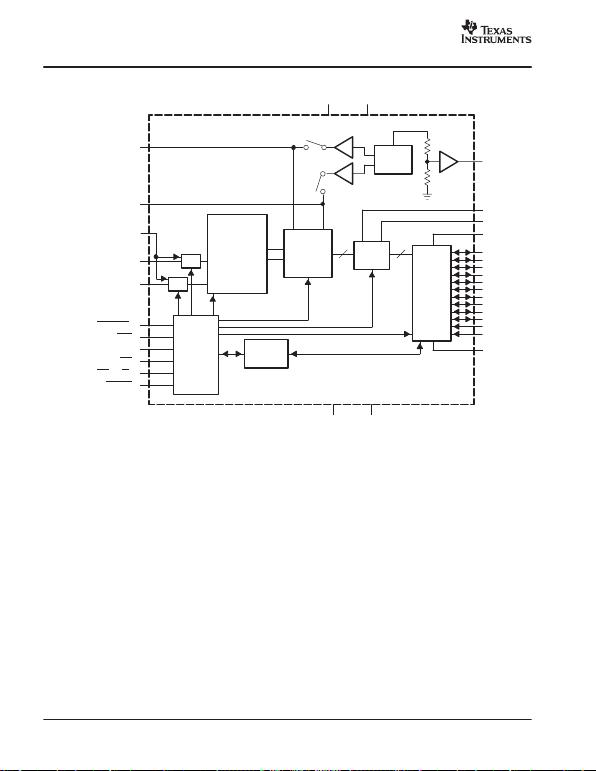
SLAS254B − M AY 2002 − REVISED NOVEMBER 2002
www.ti.com
6
FUNCTIONAL BLOCK DIAGRAM
Logic
and
Control
Control
Register
S/H
S/H
Single-Ended
and/or
Differential
MUX
10-Bit
Pipeline
REFP REFM
1.225 V
REF
2.5 V
FIFO
16 × 10
10 10
Buffers
REFOUT
DATA_AV
OV_FL
BVDD
D0
D1
D2
D3
D4
D5
D6
D7
D8
D9
RA0
RA1
BGND
AGND DGND
3.5 V
1.5 V
AV
DD
DV
DD
REFP
REFM
AINP
AINM
CONV_CLK (CONVST)
CS0
CS1
RD
WR (R/W)
RESET
REFIN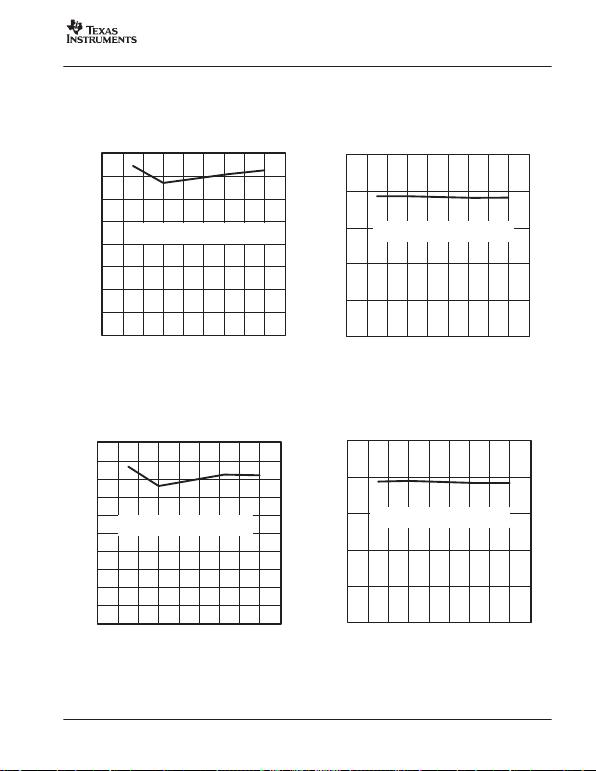
SLAS254B − M AY 2002 − REVISED NOVEMBER 2002
www.ti.com
7
TYPICAL CHARACTERISTICS
Figure 1
40
45
50
55
60
65
70
75
80
0123456789
TOTAL HARMONIC DISTORTION
vs
SAMPLING FREQUENCY (SINGLE-ENDED)
AVDD = 5 V, DVDD = BVDD = 3 V,
fIN = 500 kHz, AIN = −1 dB FS
fs − Sampling Frequency − MHz
THD − Total Harmonic Distortion − dB
Figure 2
40
45
50
55
60
65
0123456789
SIGNAL-TO-NOISE AND DIST ORTION
vs
SAMPLING FREQUENCY (SINGLE-ENDED)
fs − Sampling Frequency − MHz
SINAD − Signal-to-Noise and Distortion − dB
AVDD = 5 V, DVDD = BVDD = 3 V,
fIN = 500 kHz, AIN = −1 dB FS
Figure 3
40
45
50
55
60
65
70
75
80
85
90
0123456789
SPURIOUS FREE DYNAMIC RANGE
vs
SAMPLING FREQUENCY (SINGLE-ENDED)
fs − Sampling Frequency − MHz
SFDR − Spurious Free Dynamic Range − dB
AVDD = 5 V, DVDD = BVDD = 3 V,
fIN = 500 kHz, AIN = −1 dB FS
Figure 4
SIGNAL-TO-NOISE
vs
SAMPLING FREQUENCY (SINGLE-ENDED)
fs − Sampling Frequency − MHz
SNR − Signal-to-Noise − dB
40
45
50
55
60
65
0123456789
AVDD = 5 V, DVDD = BVDD = 3 V,
fIN = 500 kHz, AIN = −1 dB FS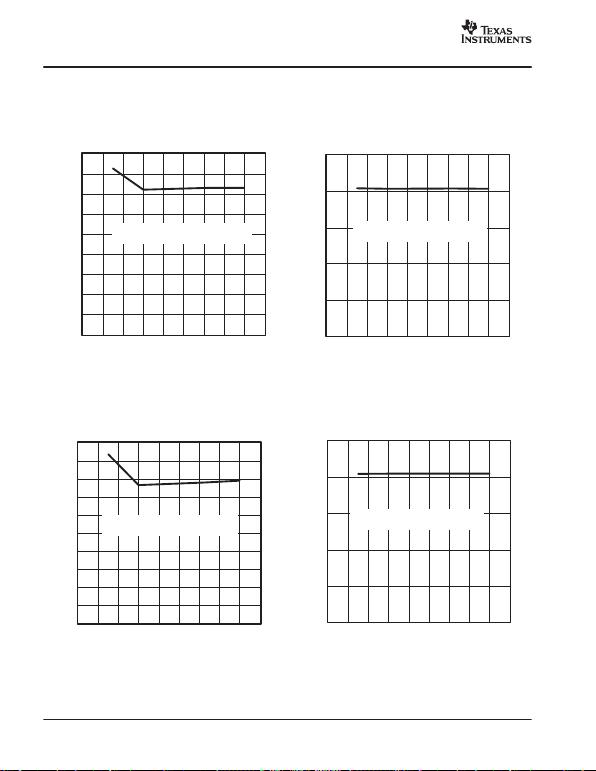
SLAS254B − M AY 2002 − REVISED NOVEMBER 2002
www.ti.com
8
TYPICAL CHARACTERISTICS
Figure 5
40
45
50
55
60
65
70
75
80
85
0123456789
TOTAL HARMONIC DISTORTION
vs
SAMPLING FREQUENCY (DIFFERENTIAL)
AVDD = 5 V, DVDD = BVDD = 3 V,
fIN = 500 kHz, AIN = −1 dB FS
fs − Sampling Frequency − MHz
THD − Total Harmonic Distortion − dB
Figure 6
40
45
50
55
60
65
0123456789
SIGNAL-TO-NOISE AND DIST ORTION
vs
SAMPLING FREQUENCY (DIFFERENTIAL)
fs − Sampling Frequency − MHz
SINAD − Signal-to-Noise and Distortion − dB
AVDD = 5 V, DVDD = BVDD = 3 V,
fIN = 500 kHz, AIN = −1 dB FS
Figure 7
40
45
50
55
60
65
70
75
80
85
90
0123456789
SPURIOUS FREE DYNAMIC RANGE
vs
SAMPLING FREQUENCY (DIFFERENTIAL)
fs − Sampling Frequency − MHz
SFDR − Spurious Free Dynamic Range − dB
AVDD = 5 V, DVDD = BVDD = 3 V,
fIN = 500 kHz, AIN = −1 dB FS
Figure 8
SIGNAL-TO-NOISE
vs
SAMPLING FREQUENCY (DIFFERENTIAL)
fs − Sampling Frequency − MHz
SNR − Signal-to-Noise − dB
40
45
50
55
60
65
0123456789
AVDD = 5 V, DVDD = BVDD = 3 V,
fIN = 500 kHz, AIN = −1 dB FS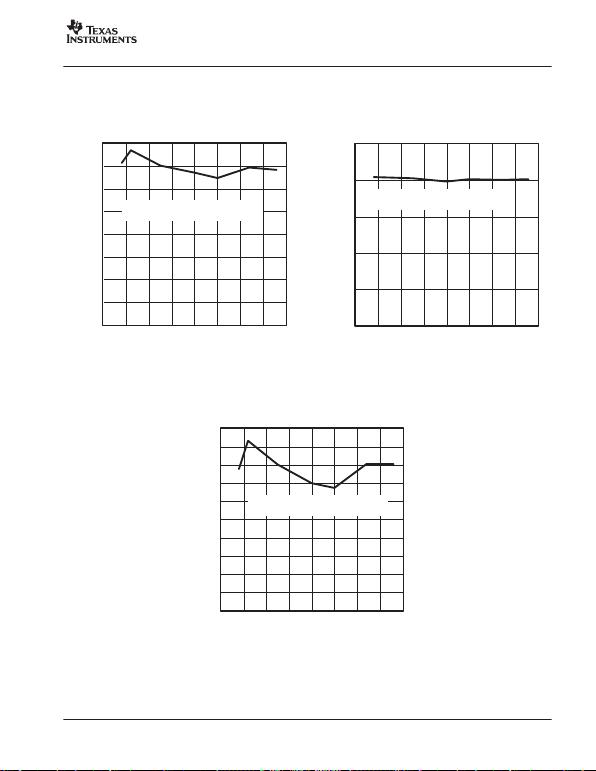
SLAS254B − M AY 2002 − REVISED NOVEMBER 2002
www.ti.com
9
TYPICAL CHARACTERISTICS
Figure 9
40
45
50
55
60
65
70
75
80
0.0 0.5 1.0 1.5 2.0 2.5 3.0 3.5 4.0
THD − Total Harmonic Distortion −dB
TOTAL HARMONIC DISTORTION
vs
INPUT FREQUENCY (DIFFERENTIAL)
AVDD = 5 V, DVDD = BVDD = 3 V,
fs = 800 MSPS, AIN = −1 dB FS
fi − Input Frequency − MHz Figure 10
40
45
50
55
60
65
0.0 0.5 1.0 1.5 2.0 2.5 3.0 3.5 4.0
SINAD − Signal-to-Noise and Distortion − dB
SIGNAL-TO-NOISE AND DIST ORTION
vs
INPUT FREQUENCY (DIFFERENTIAL)
AVDD = 5 V, DVDD = BVDD = 3 V,
fs = 8 MSPS, AIN = −1 dB FS
fi − Input Frequency − MHz
Figure 11
40
45
50
55
60
65
70
75
80
85
90
0.0 0.5 1.0 1.5 2.0 2.5 3.0 3.5 4.0
SFDR − Spurious Free Dynamic Range − dB
SPURIOUS FREE DYNAMIC RANGE
vs
INPUT FREQUENCY (DIFFERENTIAL)
AVDD = 5 V, DVDD = BVDD = 3 V,
fs = 8 MSPS, AIN = −1 dB FS
fi − Input Frequency − MHz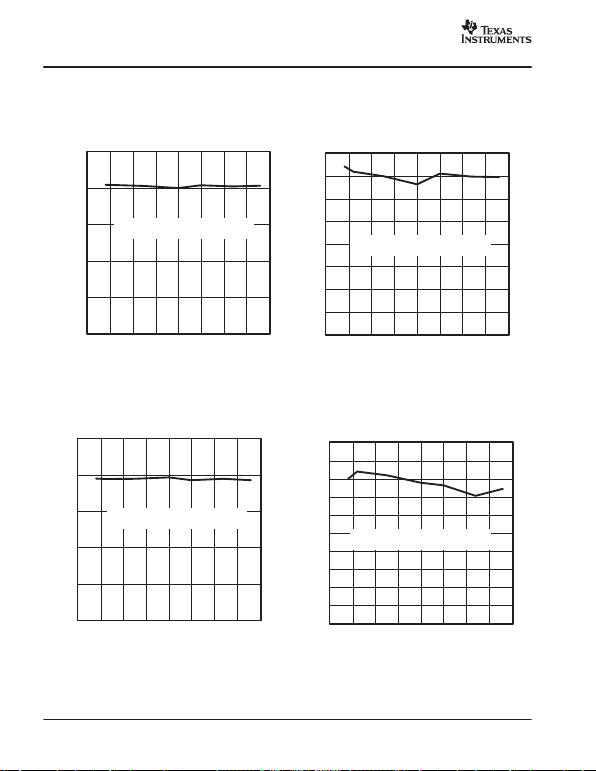
SLAS254B − M AY 2002 − REVISED NOVEMBER 2002
www.ti.com
10
TYPICAL CHARACTERISTICS
Figure 12
40
45
50
55
60
65
0.0 0.5 1.0 1.5 2.0 2.5 3.0 3.5 4.0
SNR − Signal-to-Noise − dB
SIGNAL-TO-NOISE
vs
INPUT FREQUENCY (DIFFERENTIAL)
AVDD = 5 V, DVDD = BVDD = 3 V,
fs = 8 MSPS, AIN = −1 dB FS
fi − Input Frequency − MHz Figure 13
40
45
50
55
60
65
70
75
80
0.0 0.5 1.0 1.5 2.0 2.5 3.0 3.5 4.0
TOTAL HARMONIC DISTORTION
vs
INPUT FREQUENCY (SINGLE-ENDED)
fi − Input Frequency − MHz
THD − Total Harmonic Distortion − dB
AVDD = 5 V, DVDD = BVDD = 3 V,
fs = 8 MSPS, AIN = −1 dB FS
Figure 14
40
45
50
55
60
65
0.0 0.5 1.0 1.5 2.0 2.5 3.0 3.5 4.0
SIGNAL-TO-NOISE AND DIST ORTION
vs
INPUT FREQUENCY (SINGLE-ENDED)
AVDD = 5 V, DVDD = BVDD = 3 V,
fs= 8 MSPS, AIN = −1 dB FS
fi − Input Frequency − MHz
SINAD − Signal-to-Noise and Distortion − dB
Figure 15
40
45
50
55
60
65
70
75
80
85
90
0.0 0.5 1.0 1.5 2.0 2.5 3.0 3.5 4.0
SPURIOUS FREE DYNAMIC RANGE
vs
INPUT FREQUENCY (SINGLE-ENDED)
fi − Input Frequency − MHz
AVDD = 5 V, DVDD = BVDD = 3 V,
fs = 8 MSPS, AIN = −1 dB FS
SFDR − Spurious Free Dynamic Range − dB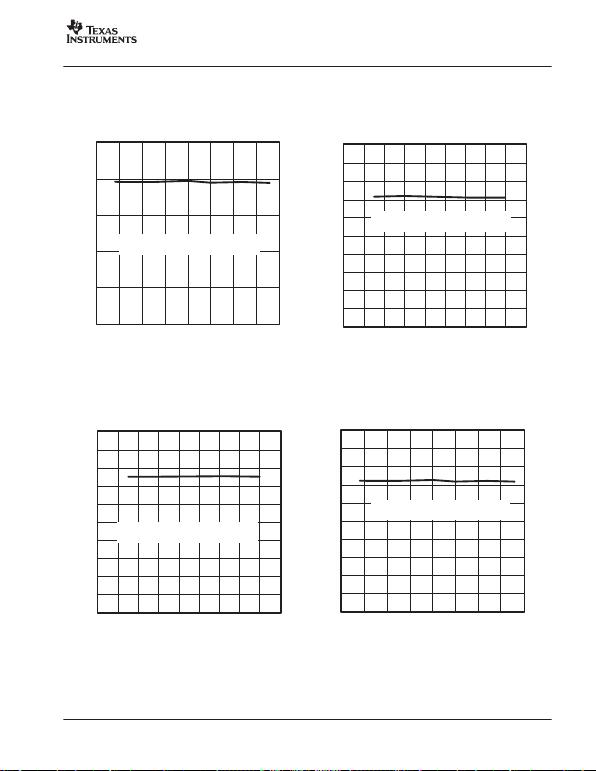
SLAS254B − M AY 2002 − REVISED NOVEMBER 2002
www.ti.com
11
TYPICAL CHARACTERISTICS
Figure 16
SIGNAL-TO-NOISE
vs
INPUT FREQUENCY (DIFFERENTIAL)
fi − Input Frequency − MHz
SNR − Signal-to-Noise − dB
40
45
50
55
60
65
0.0 0.5 1.0 1.5 2.0 2.5 3.0 3.5 4.0
AVDD = 5 V, DVDD = BVDD = 3 V,
fs = 8 MSPS, AIN = −1 dB FS
Figure 17
6.0
6.5
7.0
7.5
8.0
8.5
9.0
9.5
10.0
10.5
11.0
0123456789
ENOB − Effective Number of Bits − Bits
EFFECTIVE NUMBER OF BITS
vs
SAMPLING FREQUENCY (SINGLE-ENDED)
AVDD = 5 V, DVDD = BVDD = 3 V,
fIN = 500 kHz, AIN = −1 dB FS
fs − Sampling Frequency − MHz
Figure 18
6.0
6.5
7.0
7.5
8.0
8.5
9.0
9.5
10.0
10.5
11.0
0123456789
EFFECTIVE NUMBER OF BITS
vs
SAMPLING RATE (DIFFERENTIAL)
fs − Sampling Frequency − MHz
ENOB − Effective Number of Bits − dB
AVDD = 5 V, DVDD = BVDD = 3 V,
fIN = 500 kHz, AIN = −1 dB FS
Figure 19
6.0
6.5
7.0
7.5
8.0
8.5
9.0
9.5
10.0
10.5
11.0
0.0 0.5 1.0 1.5 2.0 2.5 3.0 3.5 4.0
EFFECTIVE NUMBER OF BITS
vs
INPUT FREQUENCY (SINGLE-ENDED)
AVDD = 5 V, DVDD = BVDD = 3 V,
fs = 8 MSPS, AIN = −1 dB FS
fi − Input Frequency − MHz
ENOB − Effective Number of Bits − dB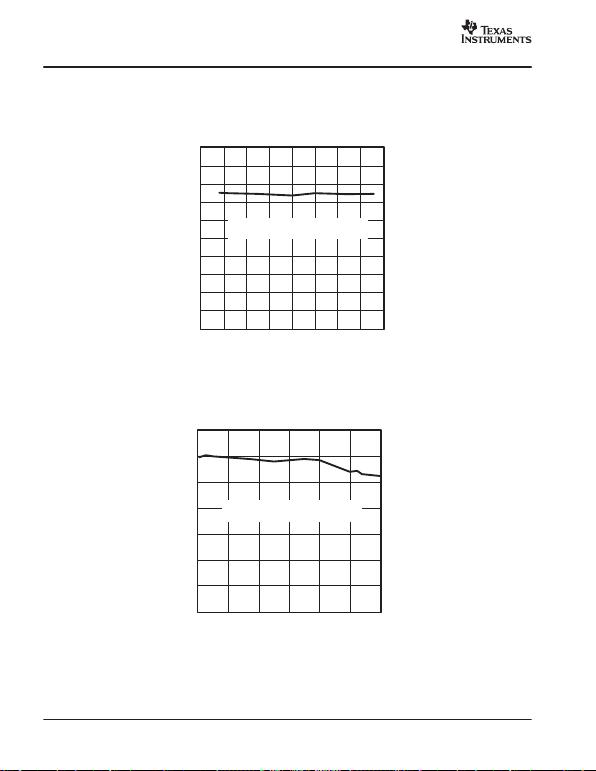
SLAS254B − M AY 2002 − REVISED NOVEMBER 2002
www.ti.com
12
TYPICAL CHARACTERISTICS
Figure 20
6.0
6.5
7.0
7.5
8.0
8.5
9.0
9.5
10.0
10.5
11.0
0.0 0.5 1.0 1.5 2.0 2.5 3.0 3.5 4.0
EFFECTIVE NUMBER OF BITS
vs
INPUT FREQUENCY (DIFFERENTIAL)
fi − Input Frequency − MHz
ENOB − Effective Number of Bits − dB
AVDD = 5 V, DVDD = BVDD = 3 V,
fs = 8 MSPS, AIN = −1 dB FS
Figure 21
GAIN
vs
INPUT FREQUENCY (SINGLE-ENDED)
fi − Input Frequency − MHz
G − Gain − dB
−30
−25
−20
−15
−10
−5
0
5
0 20406080100120
AVDD = 5 V, DVDD = BVDD = 3 V,
fs = 8 MSPS, AIN = −1 dB FS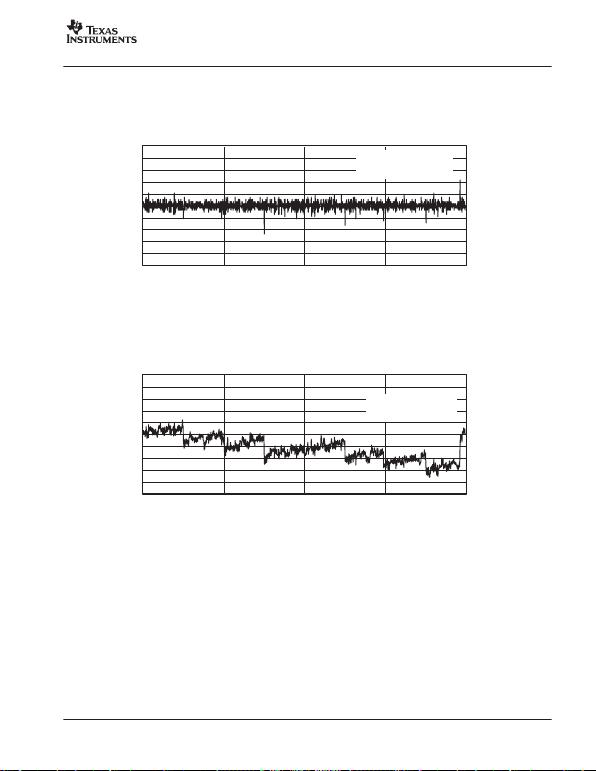
SLAS254B − M AY 2002 − REVISED NOVEMBER 2002
www.ti.com
13
TYPICAL CHARACTERISTICS
−1.0
−0.8
−0.6
−0.4
−0.2
−0.0
0.2
0.4
0.6
0.8
1.0
0 256 512 768 1024
Figure 22
DNL − Differential Nonlinearity − LSB
Code
DIFFERENTIAL NONLINEARITY
vs
AVDD = 5 V,
DVDD = BVDD = 3 V,
fs = 8 MSPS
Figure 23
−1.0
−0.8
−0.6
−0.4
−0.2
−0.0
0.2
0.4
0.6
0.8
1.0
0 256 512 768 1024
INL − Integral Nonlinearity − LSB
Code
INTEGRAL NONLINEARITY
vs
AVDD = 5 V,
DVDD = BVDD = 3 V,
fs = 8 MSPS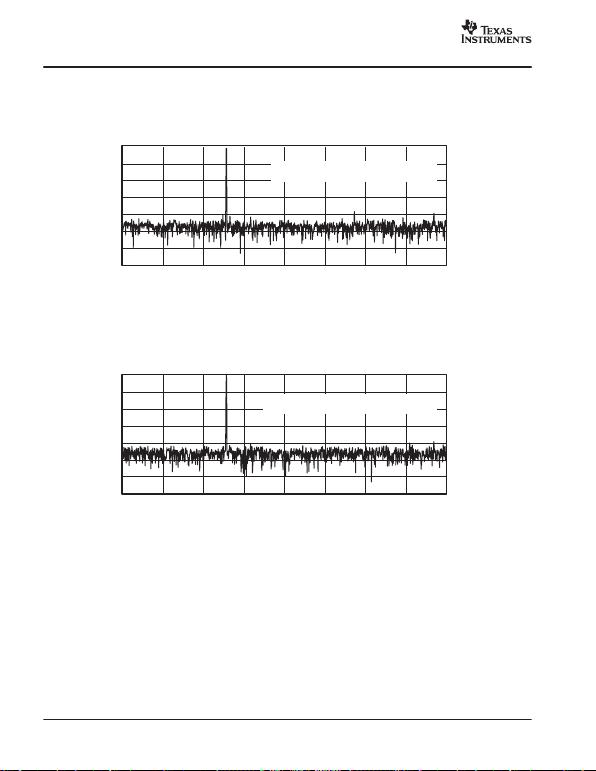
SLAS254B − M AY 2002 − REVISED NOVEMBER 2002
www.ti.com
14
TYPICAL CHARACTERISTICS
Figure 24
−140
−120
−100
−80
−60
−40
−20
0
0 500000 1000000 1500000 2000000 2500000 3000000 3500000 4000000
Magnitude − dB
f − Frequency − Hz
FAST FOURIER TRANSFORM
vs
FREQUENCY
(4096 POINTS) (SINGLE-ENDED MODE)
AVDD = 5 V, DVDD = BVDD = 3 V,
fs = 8 MHz, AIN = −1 dB FS, fIN = 1.25 MHz
Figure 25
−140
−120
−100
−80
−60
−40
−20
0
0 500000 1000000 1500000 2000000 2500000 3000000 3500000 4000000
Magnitude − dB
f − Frequency − Hz
FAST FOURIER TRANSFORM
vs
FREQUENCY
(4096 POINTS) (DIFFERENTIAL MODE)
AVDD = 5 V, DVDD = BVDD = 3 V,
fs = 8 MHz, AIN = −0.5 dB FS, fIN = 1.25 MHz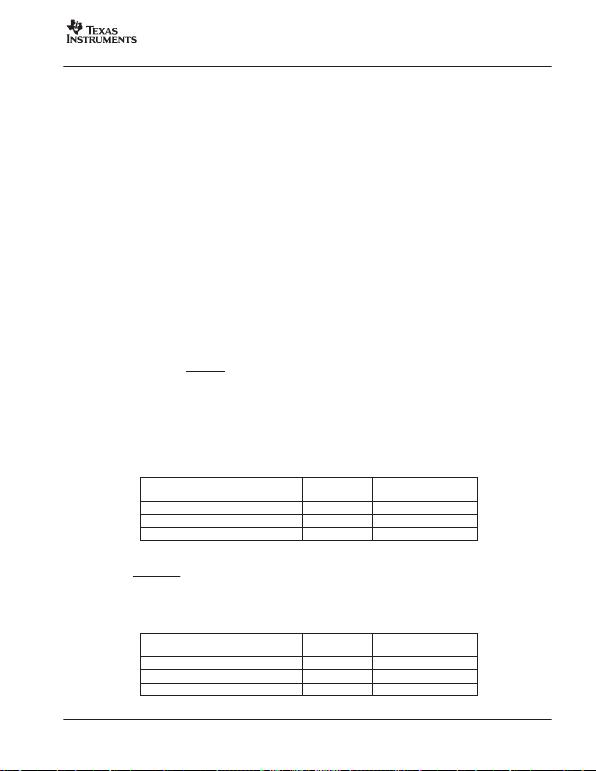
SLAS254B − M AY 2002 − REVISED NOVEMBER 2002
www.ti.com
15
DETAILED DESCRIPTION
Reference Voltage
The THS10082 has a built-in reference, which provides the reference voltages for the ADC. VREFP is set to 3.5 V and
VREFM is set to 1.5 V. An external reference can also be used through two reference input pins, REFP and REFM, if the
reference source is programmed as external. The voltage levels applied to these pins establish the upper and lower limits
The THS10082 consists of two analog inputs, which are sampled simultaneously. These inputs can be selected individually
and configured as single-ended or differential inputs. The desired analog input channel can be programmed.
Analog-to-Digital Converter
The THS10082 uses a 10-bit pipelined multistaged architecture with four 1-bit stages followed by four 2-bit stages, which
achieves a high sample rate with low power consumption. The THS10082 distributes the conversion over several smaller
ADC sub-blocks, refining the conversion with progressively higher accuracy as the device passes the results from stage
to stage. This distributed conversion requires a small fraction of the number of comparators used in a traditional flash ADC.
A sample-and-hold amplifier (SHA) within each of the stages permits the first stage to operate on a new input sample while
the second through the eighth stages operate on the seven preceding samples.
DATA_AV
In continuous conversion mode, the first DATA_A V signal is delayed by (7+TL) cycles of the CONV_CLK after a FIFO reset.
This is due to the latency of the pipeline architecture of the THS10082.
Conversion Modes
The conversion can be performed in two different conversion modes. In the single conversion mode, the conversion is
initiated by an external signal (CONVST). An internal oscillator controls the conversion time. In the continuous conversion
mode, an external clock signal is applied to the clock input (CONV_CLK). A new conversion is started with every falling
edge of the applied clock signal.
Sampling Rate
The maximum possible conversion rate per channel is dependent on the selected analog input channels. Table 1 shows
the maximum conversion rate in the continuous conversion mode for different combinations.
Table 1. Maximum Conversion Rate in Continuous Conversion Mode
CHANNEL CONFIGURATION NUMBER OF
CHANNELS MAXIMUM CONVERSION
RATE PER CHANNEL
One single-ended channel 18 MSPS
Two single-ended channels 24 MSPS
One differential channel 18 MSPS
The maximum conversion rate in the continuous conversion mode per channel, is given by:
fc +8 MSPS
# channels
Table 2 shows the maximum conversion rate in the single conversion mode.
Table 2. Maximum Conversion Rate in Single Conversion Mode(1)
CHANNEL CONFIGURATION NUMBER OF
CHANNELS MAXIMUM CONVERSION
RATE PER CHANNEL
1 single-ended channel 14 MSPS
2 single-ended channels 22.67 MSPS
1 differential channel 14 MSPS
(1) The maximum conversion rate with respect to the typical internal oscillator speed [i.e., 8 MHz × (tc/t2)].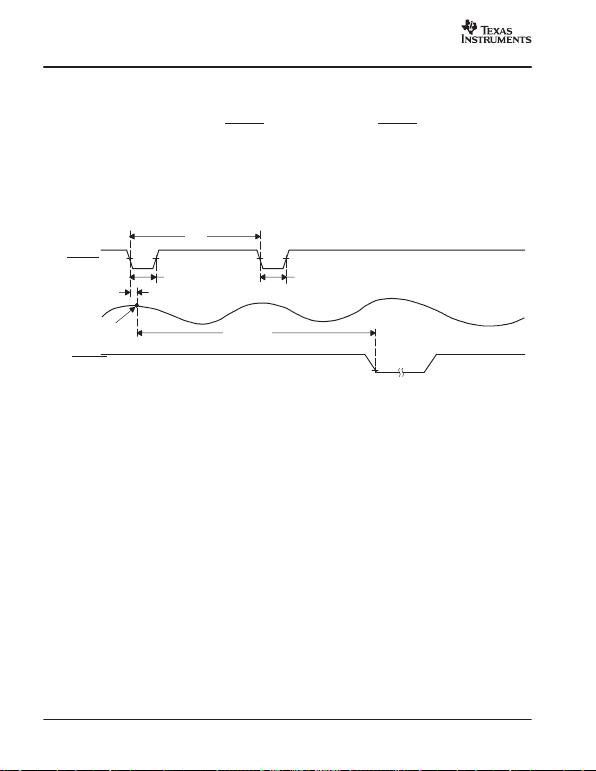
SLAS254B − M AY 2002 − REVISED NOVEMBER 2002
www.ti.com
16
SINGLE CONVERSION MODE
In single conversion mode, a single conversion of the selected analog input channels is performed. The single conversion
mode is selected by setting bit 1 of control register 0 to 1.
A single conversion is initiated by pulsing the CONVST input. On the falling edge of CONVST, the sample and hold stages
of the selected analog inputs are placed into hold simultaneously, and the conversion sequence for the selected channels
is started.
The conversion clock in single conversion mode is generated internally using a clock oscillator circuit. The signal DATA_AV
(data available) becomes active when the trigger level is reached and indicates that the converted sample(s) is (are) written
into the FIFO and can be read out. The trigger level in the single conversion mode can be selected according to Table 13.
Figure 26 shows the timing of the single conversion mode. In this mode, up to two analog input channels can be selected
to be sampled simultaneously (see Table 2).
CONVST
AIN
Sample N
t1t1
td(A)
t2
tDATA_AV
DATA_AV,
Trigger Level = 1
Figure 26. Timing of Single Conversion Mode
The time (t2) between consecutive starts of single conversions is dependent on the number of selected analog input
channels. Th e t i m e t DATA_AV, until DATA_AV becomes active is given by: tDATA_AV = tpipe + n ×tc. This equation is valid for
a trigger level which is equivalent to the number of selected analog input channels. For all other trigger level conditions refer
to the timing specifications of single conversion mode.
CONTINUOUS CONVERSION MODE
The internal clock oscillator used in the single-conversion mode is switched off in continuous conversion mode. In
continuous conversion mode, (bit 1 of control register 0 set to 0) the ADC operates with a free running external clock signal
CONV_CLK. With every rising edge of the CONV_CLK signal a new converted value is written into the FIFO.
Figure 27 shows the timing of continuous conversion mode when one analog input channel is selected. The maximum
throughput rate is 8 MSPS in this mode. The timing of the DATA_A V signal is shown here in the case of a trigger level set
to 1 or 4.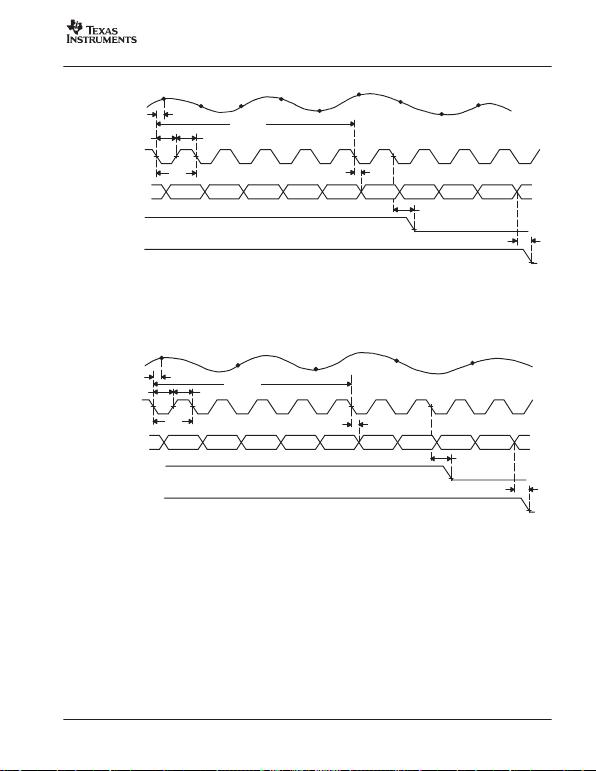
SLAS254B − M AY 2002 − REVISED NOVEMBER 2002
www.ti.com
17
Sample N
Channel 1 Sample N+1
Channel 1 Sample N+2
Channel 1 Sample N+3
Channel 1 Sample N+4
Channel 1 Sample N+5
Channel 1 Sample N+6
Channel 1 Sample N+7
Channel 1 Sample N+8
Channel 1
Data N−5
Channel 1 Data N−4
Channel 1 Data N−3
Channel 1 Data N−2
Channel 1 Data N−1
Channel 1 Data N
Channel 1 Data N+1
Channel 1 Data N+2
Channel 1 Data N+3
Channel 1
td(A)
tw(CONV_CLKH) tw(CONV_CLKL)
tctd(O)
td(DATA_AV)
td(DATA_AV)
AIN
CONV_CLK
Data Into
FIFO
DATA_AV,
Trigger Level = 1
DATA_AV,
Trigger Level = 4
td(pipe)
50% 50%
Figure 27. Timing of Continuous Conversion Mode (1-channel operation)
Figure 28 shows the timing of continuous conversion mode when two analog input channels are selected. The maximum
throughput rate per channel is 4 MSPS in this mode. The data flow in the bottom of the figure shows the order the converted
data is written into the FIFO. The timing of the DATA_AV signal shown here is for a trigger level set to 2 or 4.
AIN
CONV_CLK
Data Into
FIFO
DATA_AV,
Trigger Level = 2
DATA_AV,
Trigger Level = 4
Data N−3
Channel 2 Data N−2
Channel 1 Data N−2
Channel 2 Data N−1
Channel 1 Data N−1
Channel 2 Data N
Channel 1 Data N
Channel 2 Data N+1
Channel 1 Data N+1
Channel 2
td(DATA_AV)
tw(CONV_CLKH) tw(CONV_CLKL)
td(A)
Sample N
Channel 1,2
Sample N+1
Channel 1,2
Sample N+2
Channel 1,2
Sample N+3
Channel 1,2
Sample N+4
Channel 1,2
tctd(O)
td(Pipe)
td(DATA_AV)
50% 50%
Figure 28. Timing of Continuous Conversion Mode (2-Channel Operation)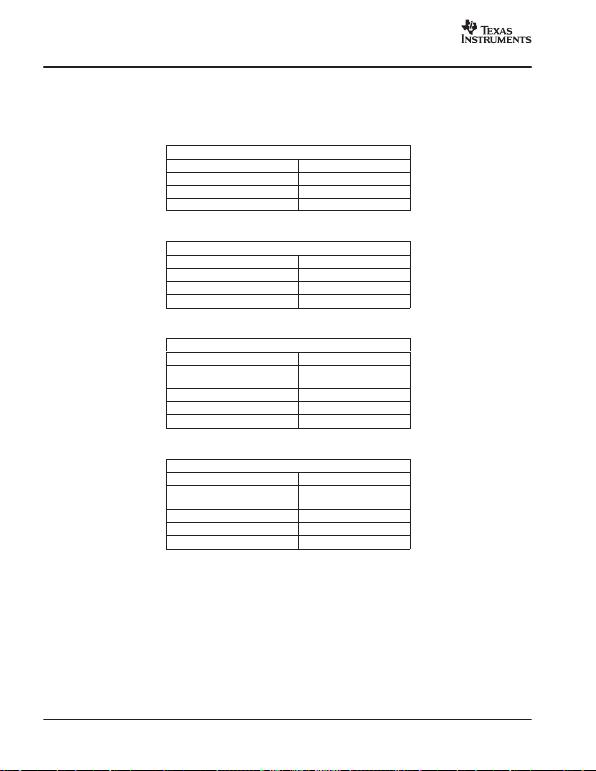
SLAS254B − M AY 2002 − REVISED NOVEMBER 2002
www.ti.com
18
DIGITAL OUTPUT DATA FORMAT
The digital output data format of the THS10082 can either be in binary format or in twos complement format. The following
Table 3. Binary Output Format for Single-Ended Configuration
SINGLE-ENDED, BINARY OUTPUT
AIN = VREFP 3FFh
AIN = (VREFP + VREFM)/2 200h
AIN = VREFM 000h
Table 4. Twos Complement Output Format for Single-Ended Configuration
SINGLE-ENDED, TWOS COMPLEMENT
AIN = VREFP 1FFh
AIN = (VREFP + VREFM)/2 000h
AIN = VREFM 200h
Table 5. Binary Output Format for Differential Configuration
DIFFERENTIAL, BINARY OUTPUT
Vin = AINP − AINM
VREF = VREFP − VREFM
Vin = VREF 3FFh
Vin = 0 200h
Vin = −VREF 000h
Table 6. Twos Complement Output Format for Differential Configuration
DIFFERENTIAL, BINARY OUTPUT
Vin = AINP − AINM
VREF = VREFP − VREFM
Vin = VREF 1FFh
Vin = 0 000h
Vin = −VREF 200h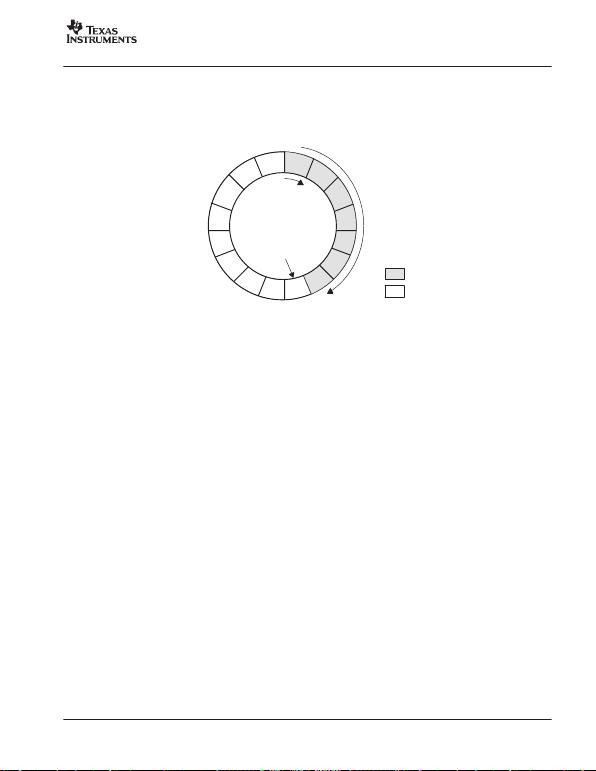
SLAS254B − M AY 2002 − REVISED NOVEMBER 2002
www.ti.com
19
FIFO DESCRIPTION
In order to facilitate an ef ficient connection to today’s processors, the THS10082 is supplied with a FIFO. This integrated
FIFO enables a problem-free processing of data with today’ s processors. The FIFO is provided as a flexible circular buffer.
The circular buffer integrated in the THS10082 stores up to 16 conversion values. Therefore, the amount of interrupts to
be served by a processor can be reduced significantly.
8
9
10
11
12
13
14
15 16 12
3
4
5
6
7
Trigger Pointer
W rite Pointer
Data in FIFO
Free
Figure 29. Circular Buffer
The converted data of the THS10082 is automatically written into the FIFO. To control the writing and reading process, a
write pointer, a read pointer and a trigger pointer are used. The read pointer always shows the location which is read next.
The write pointer indicates the location which contains the last written sample. With a selection of multiple analog input
channels, the converted values are written in a predefined sequence to the circular buffer (autoscan mode). In this way,
the channel information for the reading processor is continually maintained.
The FIFO can be programmed through the control register of the ADC. The user has the ability to select a specific trigger
level according to Table 13 in order to choose the configuration which best fits the application. The FIFO provides the signal
DATA_AV, which signals the processor to read the amount of data equal to the trigger level selected in Table 13. The signal
DATA_AV becomes active when the trigger condition is satisfied. The trigger condition is satisfied when as many values
as selected for the trigger level are written into the FIFO.
The signal DATA_AV could be connected to an interrupt input of a processor. In every interrupt service routine call, the
processor must read the amount of data equal to the trigger level from the ADC. The first data represents the first channel
according to the autoscan mode, which is shown in Table 10. The channel information is, therefore, always maintained.